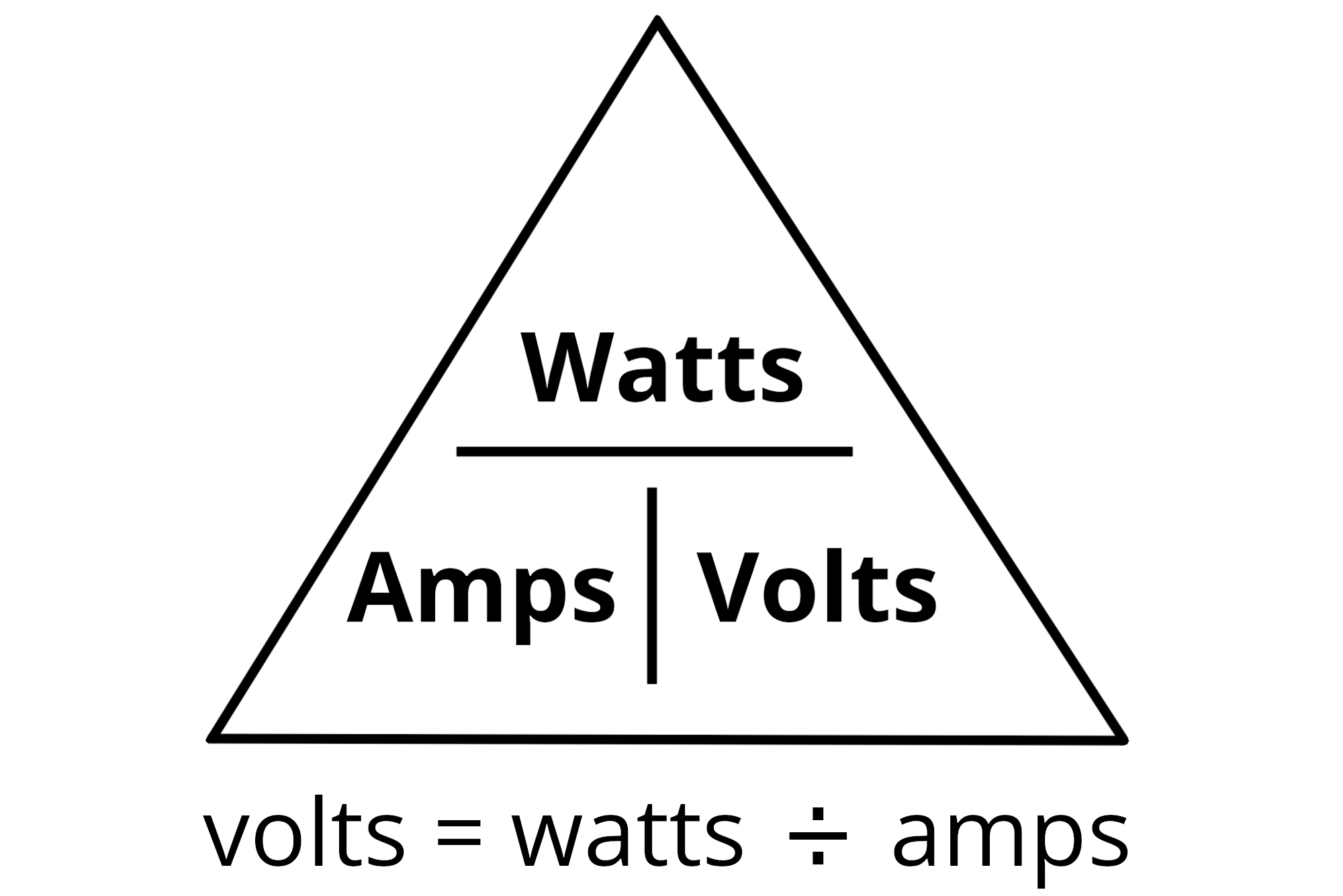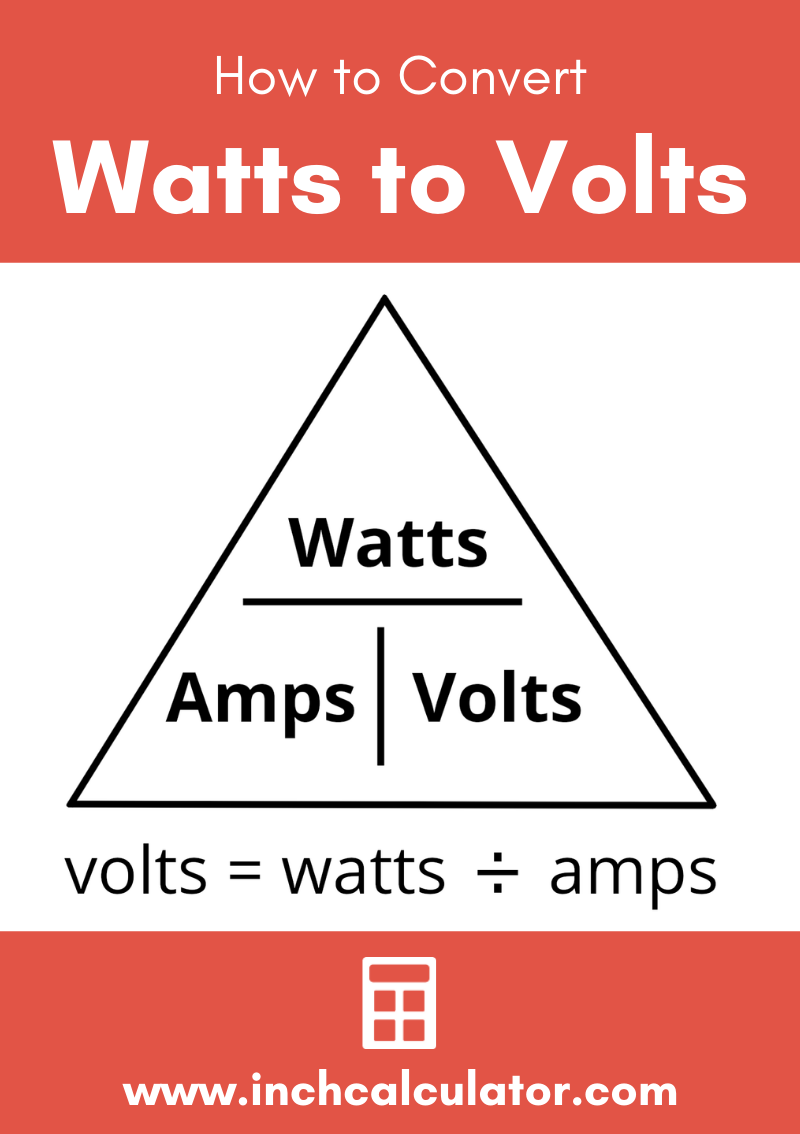# Watts to Volts Conversion Calculator

Convert watts to volts by entering the wattage and current in amps or the circuit resistance below.

## Results:

Volts
Learn how we calculated this below

## How to Convert Watts to Volts

Watts can be converted to volts using current and the Watt’s Law power formula, which states that current is equal to power divided by voltage. We can change this formula around by multiplying both sides by the voltage and restating it as the voltage is equal to the power divided by the current.

### Watts to Volts Formula

Thus, the following formula to convert wattage to voltage is derived:

V(V) = P(W) / I(A)

The voltage V in volts is equal to the power P in watts divided by the current I in amps. We can use this formula to solve for voltage by dividing the wattage by the amperage.For example, let’s convert 20 watts to volts using a DC circuit with 4 amps of current.

Voltage(V) = 20 W ÷ 4 A
Voltage(V) = 5 volts

## Conversion For AC Circuits

Converting real wattage to RMS voltage on an AC circuit is pretty similar to a DC circuit, with a slight change to account for the power factor of an AC circuit.

V(V) = P(W) / I(A) × PF

For an AC circuit, the voltage V in volts is equal to the real power P in watts divided by the product of the current I in amps and the power factor.

For example, let’s convert 1,300 watts to voltage for an AC electrical circuit with 12 amps of current and a power factor of 0.9.

V(V) = 1,300 W ÷ (12 A × 0.9)
V(V) = 120.4 volts

In this example, 1,300 watts is equal to 120.4 volts at 12 amps.

## Convert Watts to Volts using Resistance

You can also convert watts to volts if the resistance of the circuit is known. Voltage is equal to the square root of the wattage times the resistance in ohms.

V(V) = √(P(W) × R(Ω))

So, the voltage V in volts is equal to the square root of the power P in watts times the resistance R in ohms.

For example, let’s convert 40 watts to volts for a DC circuit with 10 ohms of resistance.

V(V) = √(40 W × 10 Ω)
V(V) = √400
V(V) = 20 volts

## Watts to Volts Conversion Chart

Chart showing the power in watts converted to volts at various current ratings.
Power Voltage Current
5 watts 5 volts 1 amp
5 watts 2.5 volts 2 amps
5 watts 1.667 volts 3 amps
5 watts 1.25 volts 4 amps
10 watts 10 volts 1 amp
10 watts 5 volts 2 amps
10 watts 3.333 volts 3 amps
10 watts 2.5 volts 4 amps
15 watts 15 volts 1 amp
15 watts 7.5 volts 2 amps
15 watts 5 volts 3 amps
15 watts 3.75 volts 4 amps
20 watts 20 volts 1 amp
20 watts 10 volts 2 amps
20 watts 6.667 volts 3 amps
20 watts 5 volts 4 amps
25 watts 25 volts 1 amp
25 watts 12.5 volts 2 amps
25 watts 8.333 volts 3 amps
25 watts 6.25 volts 4 amps
30 watts 30 volts 1 amp
30 watts 15 volts 2 amps
30 watts 10 volts 3 amps
30 watts 7.5 volts 4 amps
35 watts 35 volts 1 amp
35 watts 17.5 volts 2 amps
35 watts 11.667 volts 3 amps
35 watts 8.75 volts 4 amps
40 watts 40 volts 1 amp
40 watts 20 volts 2 amps
40 watts 13.333 volts 3 amps
40 watts 10 volts 4 amps
45 watts 45 volts 1 amp
45 watts 22.5 volts 2 amps
45 watts 15 volts 3 amps
45 watts 11.25 volts 4 amps
50 watts 50 volts 1 amp
50 watts 25 volts 2 amps
50 watts 16.667 volts 3 amps
50 watts 12.5 volts 4 amps
55 watts 55 volts 1 amp
55 watts 27.5 volts 2 amps
55 watts 18.333 volts 3 amps
55 watts 13.75 volts 4 amps
60 watts 60 volts 1 amp
60 watts 30 volts 2 amps
60 watts 20 volts 3 amps
60 watts 15 volts 4 amps
65 watts 65 volts 1 amp
65 watts 32.5 volts 2 amps
65 watts 21.667 volts 3 amps
65 watts 16.25 volts 4 amps
70 watts 70 volts 1 amp
70 watts 35 volts 2 amps
70 watts 23.333 volts 3 amps
70 watts 17.5 volts 4 amps
75 watts 75 volts 1 amp
75 watts 37.5 volts 2 amps
75 watts 25 volts 3 amps
75 watts 18.75 volts 4 amps
80 watts 80 volts 1 amp
80 watts 40 volts 2 amps
80 watts 26.667 volts 3 amps
80 watts 20 volts 4 amps
85 watts 85 volts 1 amp
85 watts 42.5 volts 2 amps
85 watts 28.333 volts 3 amps
85 watts 21.25 volts 4 amps
90 watts 90 volts 1 amp
90 watts 45 volts 2 amps
90 watts 30 volts 3 amps
90 watts 22.5 volts 4 amps
95 watts 95 volts 1 amp
95 watts 47.5 volts 2 amps
95 watts 31.667 volts 3 amps
95 watts 23.75 volts 4 amps
100 watts 100 volts 1 amp
100 watts 50 volts 2 amps
100 watts 33.333 volts 3 amps
100 watts 25 volts 4 amps

You might also be interested in our volts to watts calculator.## References

1. Miller, C., NFPA's Electrical References, National Fire Protection Association, 2004, Jones & Bartlett Learning, 67-75. https://www.google.com/books/edition/NFPA_s_Electrical_References/raUyIi7i-asC
2. Miller, C., Ugly’s Electrical References, 2020 Edition, 2020, Jones & Bartlett Learning, 16-23. https://books.google.com/books?id=1kS8DwAAQBAJ